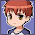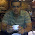## Thursday, May 17, 2012

### Different ways to print the next few lines after pattern match

grep, awk  or a sed command is used to print the line matching a particular pattern. However,  at times, we need to print a few more lines following the lines matching the pattern. In this article, we will see the different ways in which we can get this done. The first part explains ways to print one line following the pattern along with the pattern, few examples to print only the line following the pattern and in the end we have ways to print multiple lines following the pattern.

Let us consider a file with the following contents as shown below:
```\$ cat file
Unix
Linux
Solaris
AIX
SCO
```
1. The simplest is using the grep command. In GNU grep, there is an option -A which prints lines following the pattern.
```\$ grep -A1 Linux file
Linux
Solaris
```
In the above example, -A1 will print one line following the pattern along with the line matching the pattern. To print 2 lines after the pattern, it is -A2.

2. sed  has the N command which will read the next line into the pattern space.
```\$ sed -n '/Linux/{N;p}' file
Linux
Solaris
```
First, the line containing the pattern /Linux/ is found. The command within the braces will run on the pattern found. {N;p} means read the next line and print the pattern space which now contains the current and the next line. Similarly, to print 2 lines, you can simply put: {N;N;p}. Example 7 onwards explains for printing multiple lines following the pattern.

3. awk has the getline command which reads the next line from the file.
```\$ awk '/Linux/{print;getline;print;}' file
Linux
Solaris
```
Once the line containing the pattern Linux is found, it is printed. getline command reads the next line into \$0. Hence, the second print statement prints the next line in the file.

4. In this, the same thing is achieved using only one print statement.
```\$ awk '/Linux/{getline x;print \$0 RS x;}' file
Linux
Solaris
```
getline x reads the next line into variable x. x is used in order to prevent the getline from overwriting the current line present in \$0. The print statement prints the current line(\$0), record separator(RS) which is the newline, and the next line which is in x.

5. To print only the line following the pattern without the line matching the pattern:
```\$ sed  -n '/Linux/{n;p}' file
Solaris
```
The n command reads the next line into the pattern space thereby overwriting the current line. On printing the pattern space using the p command, we get the next line printed.

6. Same using awk:
```\$ awk '/Linux/{getline;print;}' file
Solaris
```
Multiple lines after the pattern:
GNU grep may not available in every Unix box. Excluding grep option, the above examples are good only to print a line or two following the pattern. Say, if you have to print some 10 lines after the pattern, the command will get clumsy. Let us now see  how to print n lines following the pattern along with the pattern:

7. To print multiple(2) lines following the pattern using awk:
```\$ awk '/Linux/{x=NR+2}(NR<=x){print}' file
Linux
Solaris
Aix
```
To print 5 lines after the pattern, simply replace the number 2 with 5. This above example is a little tricky. Once the pattern Linux is found, x is calculated which is current line number(NR) plus 2. So, we will print lines from the current line till the line number(NR) reaches x.

8. To print 2 lines following the pattern without the line matching the pattern:
```\$ awk '/Linux/{x=NR+2;next}(NR<=x){print}' file
Solaris
Aix
```
The next command makes the current line, which is the pattern matched, to get skipped. In this way, we can exclude the line matching the pattern from getting printed.

9. To print 2 lines following the pattern along with the pattern matching line in another way.
```\$ x=`grep  -n Linux file | cut -f1 -d:`
\$ awk -v ln=\$x 'NR>=ln &&  NR<=ln+2' file
```
Using grep and cut command, the line number of the pattern in the file is found. By passing the shell variable to awk,  we make it print only those lines whose line number is between  x and x+2.

10. One more way using sed and awk combination. First we calculate the from and to line numbers in the variables x and y. Using sed printing range of lines, we can get the same. sed can not only deal with numbers, it can work with variables as well:
```\$ x=`awk '/Linux/{print NR}' file`
\$ y=`awk '/Linux/{print NR+2}' file`
\$ sed -n "\$x,\$y p" file```
OR
```\$ x=`awk '/Linux/{print NR+2}' file`
\$ sed -n "/Linux/,\$x p" file
```1.tricky and useful!

2.grep -A1 filename
Command doesnt work on Solaris

1.-A option is available in GNU grep. You will find this Linux and its flavors.

2.Thanks for this, solved a problem for me :-)

3.Very helpful. Thanks to share.

How can we print next line to only first matched line. I don't want need to search all matched lines in file

For e.g.
cat file
Unix
Linux
Solaris
AIX SCO
Linux
Redhat

above command prints:
Solaris
Redhat

But I need only next line to first matching line (not to go for all rest of matched line) : i.e.
Solrais

1.Just add an exit at the end:

awk '/Linux/{getline;print;exit}' file

4.How to print all lines (till the end of the file) after the match?